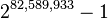# Mersenne prime facts for kids

Kids Encyclopedia Facts

In mathematics, a Mersenne number is a number that is one less than a power of two.

Mn = 2n − 1.

A Mersenne prime is a Mersenne number that is a prime number. This however, is not sufficient. Many mathematicians prefer the definition of a Mersenne number where exponent n has to be a prime number.

For example, 31 = 25 − 1, and 5 is a prime number, so 31 is a Mersenne number; and 31 is also a Mersenne prime because it is a prime number. But the Mersenne number 2047 = 211 − 1 is not a prime because it is divisible by 89 and 23. And 24 − 1 = 15 can be shown to be composite because 4 is not prime.

Throughout modern times, the largest known prime number has very often been a Mersenne prime. Most sources restrict the term Mersenne number to where n is prime, as all Mersenne primes must be of this form as seen below.

Mersenne primes have a close connection to perfect numbers, which are numbers equal to the sum of their proper divisors. Historically, the study of Mersenne primes was motivated by this connection; in the 4th century BC Euclid demonstrated that if M is a Mersenne prime then M(M+1)/2 is a perfect number. In the 18th century, Leonhard Euler proved that all even perfect numbers have this form. No odd perfect numbers are known, and it is suspected that none exist (If it exist, it would have to be greater than 101500).

It is currently unknown if there is an infinite number of Mersenne primes. Most mathematicians who have studied the question think there are an infinite number of Mersenne primes. Carl Pomerance, Samuel S. Wagstaff Jr. and Hendrik Lenstra developed a formula which they think should say about how many Mersenne primes there are under a given number.

The binary representation of 2n - 1 is n repetitions of the digit 1.

For example, 25 - 1 = 11111 in binary.

The first four Mersenne primes were known by the Ancient Greeks. They are 3, 7, 31 and 127. The next, 8191, was discovered in 1456. Some people like trying to find big primes. Since there are tricks and algorithms to finding Mersenne primes quickly, the largest known primes are also Mersenne primes.

As of December 21, 2018, 51 Mersenne primes have been found. The largest known prime is a Mersenne prime,$2^{82,589,933} - 1$.In Spanish: Número primo de Mersenne para niños